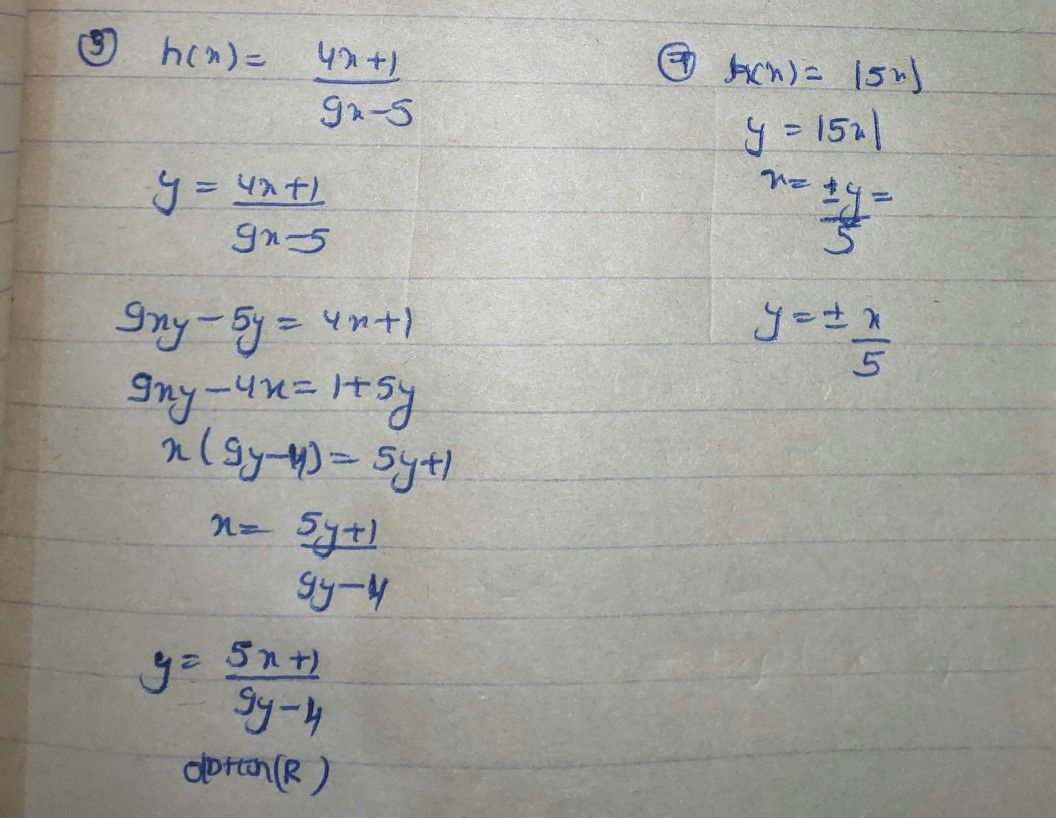Symbol
ProblemLesson Solving $Rea1-1if_{e}$ Problems 1 Involving Inverse Functions You have learned from your previous modules the representations inverse functions through its table of values, graphs, and equations. You also learned how to find its domain and range which are important in the study of solving $rca1-lif$ problems involving inverse functions. This module will help you solve $rca1-lif_{9}$ problems involving inverse functions. What's In Let us start your journey by recalling the previous lessons you already learned about inverse functions. Here is the list of functions and its inverse, match column B to colurmn A by finding the inverse of the items in column A. Write the letter of the answer in the box below that will reveal a "word" or the name of the "building" that you are looking for. The United Arab Emirates was given the title of "The Tallest Building in the World" on January $y4,2010$ What is the name of the building? Column A Column B 1. g(x) $=x5-3$ R. y = 5x+1 9x-4 2. fix) $=7x+10$ A. y 3 $3.$ h(x) = 49xx+-5 1 A. y t. x2 4. $k=\dfrac {5} {9}\left(t-32\right)+273.15$ J. $y=-\left(k-273.15$ $5$ $-273.15\right)+32$ 5. $w\left(x\right)=2x+9$ L. y 3 x+7 6. K. x-9 2 $t\left(x\right)=\dfrac {x+2} {3x-5}$ $r\left(x\right)=|5x|$ $s\left(x\right)=2x^{3-7}$ B. y = $\sqrt{x+3}$ 789. . . H. y = 5x+2 3x-1 $q\left(x\right)=3x-5$ $F.y=$ x-11 $10.n\left(x\right)=5x+11$ 11. $z\left(x\right)=3t$ R. $1.y=\dfrac {x+5} {3}$ $y=\sqrt{\dfrac {x-2} {7}}$ $y=\dfrac {x-10} {7}$ U.
10th-13th grade
Algebra
Search count: 115
Question content
Pleasee help me to solve this step by step please maam thankyou
SolutionQanda teacher - AskhinaStudent
Thankyou maam ❤️